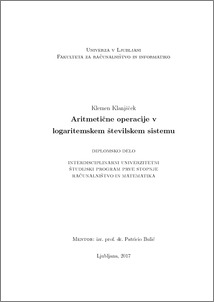# Logarithmic Number System Based Arithmetic Operations

Klemen Klanjšček (2017) Logarithmic Number System Based Arithmetic Operations. EngD thesis.Preview
PDF

## Abstract

In this thesis, we discuss the logarithmic number system and its applications as an alternative to FP. We were interested in its binary representation, logical implementation of arithmetic and the numerical errors associated with it, and the arguments we can make in its favor. In particular, we focused on 16-bit LNS in base e. Using the theory of uniform approximation, a model was built for the approximation of the Gaussian logarithms needed to add and subtract in LNS. Finally, the arithmetic unit in 16-bit LNS in base e was implemented in FPGA. Assuming there is an efficient LNS to FP (and vice versa) conversion method available, we believe our implementations have applications in numerically demanding systems that do not require a high degree of accuracy in their calculations.

Item Type: Thesis (EngD thesis)
Keywords: arithmetic, LNS, FP, polynomial, approximation, uniform approximation, Remez algorithm, digital design, FPGA
Number of Pages: 79
Language of Content: Slovenian
Mentor / Comentors:
Name and SurnameIDFunction
izr. prof. dr. Patricio Bulić255Mentor